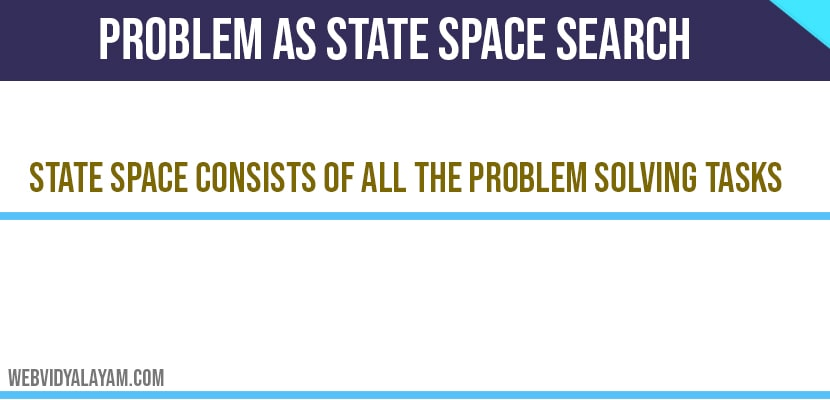# Problem As State Space Search

State space consists of all the problem solving tasks.

State space: A state space consists of all the states ,set of operators that shifts  from one state to another.

States are also known as nodes in a connected graph and the edges are denoted as operators. Problem solving can be formulated as search in state space. For example to find the solution of an 8 puzzle game which is represented as 3*3 matrix.

One of the tile is unoccupied by moving this tile to other positions we can create new states. For example if I move this unoccupied block to right then it becomes

New state

Here we can create ‘n’ number of states. The number of new states generated all together constitutes to a state space.

All of these states of the domain and all of these operators. In this case it could be 4 operators they are the tile can move towards right, left ,up and down. All these operators together constitute to asset of operators. Once the set of operators and actions are defined then move on to finding solution.

For suppose there are two states start state and end state. If the path from start state to end state is found then the problem is solved. State space is defined as the set of all possible configurations that one get from the relevant objects. This is also referred to problem space.

Let us take previous example 3*3 matrix having 8 elements and one empty space. This empty space is a problem state. Suppose the first matrix is start and the second is destination, the path from ‘S’ to ’D’ the total number of configurations is 9!.

Start state ‘S’

Destination State ‘D’